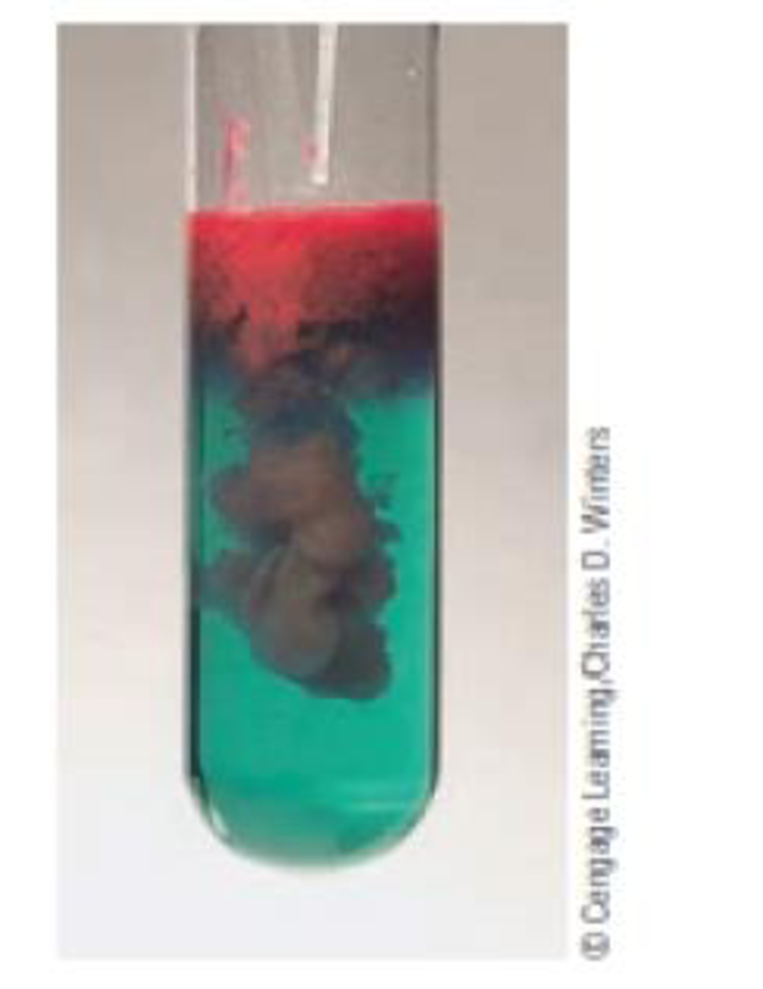# A common method for analyzing for the nickel content of a sample is to use a precipitation reaction. Adding the organic compound dimethylgly-oxime to a solution containing Ni 2+ ions precipitates a red solid. Derive the empirical formula for the red solid based on the following composition: Ni, 20.315%; C, 33.258%; H, 4.884%; 0, 22.151%; and N, 19.392%.### Chemistry & Chemical Reactivity

9th Edition
John C. Kotz + 3 others
Publisher: Cengage Learning
ISBN: 9781133949640

#### Solutions

Chapter
Section### Chemistry & Chemical Reactivity

9th Edition
John C. Kotz + 3 others
Publisher: Cengage Learning
ISBN: 9781133949640
Chapter 3, Problem 91SCQ
Textbook Problem
34 views

## A common method for analyzing for the nickel content of a sample is to use a precipitation reaction. Adding the organic compound dimethylgly-oxime to a solution containing Ni2+ ions precipitates a red solid.Derive the empirical formula for the red solid based on the following composition: Ni, 20.315%; C, 33.258%; H, 4.884%; 0, 22.151%; and N, 19.392%.

Interpretation Introduction

Interpretation:

The empirical formula for the red solid based on the given composition should be derived.

Concept introduction:

Get the mass of each element by assuming a certain overall mass for the sample.  Convert the mass of each element to moles of each element using the atomic masses.  Find the ratio or the moles of each element by dividing the number of moles of each by the smallest number of moles.

### Explanation of Solution

The molar mass of carbon is 12.00, the molar mass of nickel is 58.7, the molar mass of hydrogen is 1.00, the molar mass of oxygen is 16.0 and the molar mass of nitrogen is 14.0.

Therefore the empirical formula of the red solid is calculated below,

33.285 g ×1Molarmassofcarbon33.285 g ×112.00=2.7715

20.315 g ×1Molarmassofnickel20.315 g ×158.7=0.346

4.884 g ×1Molarmassofhydrogen4.884 g ×11=4.884

22.121 g ×1Molarmassofoxygen4

### Still sussing out bartleby?

Check out a sample textbook solution.

See a sample solution

#### The Solution to Your Study Problems

Bartleby provides explanations to thousands of textbook problems written by our experts, many with advanced degrees!

Get Started

Find more solutions based on key concepts
Characterize gases, liquids, and solids in terms of compressibility and fluidity.

General Chemistry - Standalone book (MindTap Course List)

One way to evaluate any diet is to compare the total food amounts that it provides with those recommended by th...

Nutrition: Concepts and Controversies - Standalone book (MindTap Course List)

A cars speedometer reads instantaneous ___. (2.2)

An Introduction to Physical Science

Why are buffers important for living organisms?

Biology: The Dynamic Science (MindTap Course List)

In what way has biotechnology had an impact on agriculture in the United States?

Human Heredity: Principles and Issues (MindTap Course List)

The liquid-drop model of the atomic nucleus suggests high-energy oscillations of certain nuclei can split the n...

Physics for Scientists and Engineers, Technology Update (No access codes included)

Satellites orbit in space. How can a satellite conduct oceanography research?

Oceanography: An Invitation To Marine Science, Loose-leaf Versin

Why do you crumple up a sheet of paper before you toss it toward the garbage can?

Physics for Scientists and Engineers: Foundations and Connections